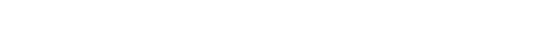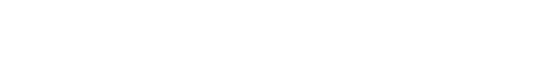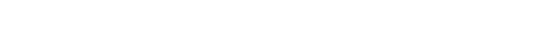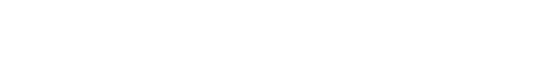XGBoost也可以用于时间序列预测，尽管要先把时间序列数据集转换成适用于有监督学习的形式。它还需要使用一种专门的技术来评估模型，称为前向推进验证，因为模型评估使用了k-折叠交叉，这会产生有正偏差的结果。

XGBoost是用于分类和回归问题的梯度提升集成方法的一个实现。

#### 一、XGBoost集成

Tree boosting has been shown to give state-of-the-art results onmany standard classification benchmarks.

— XGBoost:A Scalable Tree Boosting System, 2016.

https://arxiv.org/abs/1603.02754

XGBoost是随机梯度提升算法的一种高效实现，它可以通过一系列模型超参数在整个训练过程中控制模型。

The mostimportant factor behind the success of XGBoost is its scalability in allscenarios. The system runs more than ten times faster than existing popularsolutions on a single machine and scales to billions of examples in distributedor memory-limited settings.

— XGBoost: A Scalable TreeBoosting System, 2016.

https://arxiv.org/abs/1603.02754

XGBoost是为表格式数据集的分类和回归问题而设计的，也可以用于时间序列预测。

《机器学习中梯度提升算法的简要概括》#### 二、时间序列数据准备《Time Series Forecasting as Supervised Learning》我们可以使用此函数为XGBoost准备一个时间序列数据集。

《如何在Python中将时间序列转化为监督学习问题》

《How To Backtest Machine Learning Models for Time Series Forecasting》train_test_split()函数是用来把数据集划分为训练集和测试集的。可以如下定义这个方法：#### 三、XGBoost用于时间序列预测

Dataset (daily-total-female-births.csv)

Description (daily-total-female-births.names)# forecast monthlybirths with xgboost

from numpy importasarray

from pandas importDataFrame

from pandas importconcat

from sklearn.metricsimport mean_absolute_error

from xgboost importXGBRegressor

from matplotlib importpyplot

# transform a timeseries dataset into a supervised learning dataset

defseries_to_supervised(data, n_in=1, n_out=1, dropnan=True):

n_vars = 1 if type(data) is list elsedata.shape

df = DataFrame(data)

cols = list()

# input sequence (t-n, … t-1)

for i in range(n_in, 0, -1):

cols.append(df.shift(i))

# forecast sequence (t, t+1, … t+n)

for i in range(0, n_out):

cols.append(df.shift(-i))

# put it all together

agg = concat(cols, axis=1)

# drop rows with NaN values

if dropnan:

agg.dropna(inplace=True)

return agg.values

# split a univariatedataset into train/test sets

deftrain_test_split(data, n_test):

return data[:-n_test, :], data[-n_test:,:]

# fit an xgboost modeland make a one step prediction

def xgboost_forecast(train,testX):

# transform list into array

train = asarray(train)

# split into input and output columns

trainX, trainy = train[:, :-1], train[:,-1]

# fit model

model =XGBRegressor(objective=’reg:squarederror’, n_estimators=1000)

model.fit(trainX, trainy)

# make a one-step prediction

yhat = model.predict(asarray([testX]))

return yhat

# walk-forwardvalidation for univariate data

defwalk_forward_validation(data, n_test):

predictions = list()

# split dataset

train, test = train_test_split(data,n_test)

# seed history with training dataset

history = [x for x in train]

# step over each time-step in the testset

for i in range(len(test)):

# split test row into input andoutput columns

testX, testy = test[i, :-1],test[i, -1]

# fit model on history and make aprediction

yhat = xgboost_forecast(history,testX)

# store forecast in list ofpredictions

predictions.append(yhat)

# add actual observation tohistory for the next loop

history.append(test[i])

# summarize progress

print(‘>expected=%.1f,predicted=%.1f’ % (testy, yhat))

# estimate prediction error

error = mean_absolute_error(test[:, 1],predictions)

return error, test[:, 1], predictions

values = series.values

# transform the timeseries data into supervised learning

data =series_to_supervised(values, n_in=3)

# evaluate

mae, y, yhat =walk_forward_validation(data, 12)

print(‘MAE: %.3f’ %mae)

# plot expected vspreducted

pyplot.plot(y,label=’Expected’)

pyplot.plot(yhat,label=’Predicted’)

pyplot.legend()

pyplot.show()# finalize model andmake a prediction for monthly births with xgboost

from numpy importasarray

from pandas importDataFrame

from pandas importconcat

from xgboost importXGBRegressor

# transform a timeseries dataset into a supervised learning dataset

defseries_to_supervised(data, n_in=1, n_out=1, dropnan=True):

n_vars = 1 if type(data) is list elsedata.shape

df = DataFrame(data)

cols = list()

# input sequence (t-n, … t-1)

for i in range(n_in, 0, -1):

cols.append(df.shift(i))

# forecast sequence (t, t+1, … t+n)

for i in range(0, n_out):

cols.append(df.shift(-i))

# put it all together

agg = concat(cols, axis=1)

# drop rows with NaN values

if dropnan:

agg.dropna(inplace=True)

return agg.values

values = series.values

# transform the timeseries data into supervised learning

train =series_to_supervised(values, n_in=3)

# split into input andoutput columns

trainX, trainy =train[:, :-1], train[:, -1]

# fit model

model =XGBRegressor(objective=’reg:squarederror’, n_estimators=1000)

model.fit(trainX,trainy)

# construct an inputfor a new preduction

row = values[-3:].flatten()

# make a one-stepprediction

yhat =model.predict(asarray([row]))

print(‘Input: %s,Predicted: %.3f’ % (row, yhat))

#### 进一步阅读

A Gentle Introduction to the Gradient Boosting Algorithm for Machine LearningTime Series Forecasting as Supervised LearningHow to Convert a Time Series to a Supervised Learning Problem in PythonHow To Backtest Machine Learning Models for Time Series     Forecasting

How To Backtest Machine Learning Models for Time Series Forecasting#### 总结

XGBoost是用于分类和回归的梯度boosting集成算法的实现

How to Use XGBoost for Time Series Forecasting

How to Use XGBoost for Time Series Forecasting